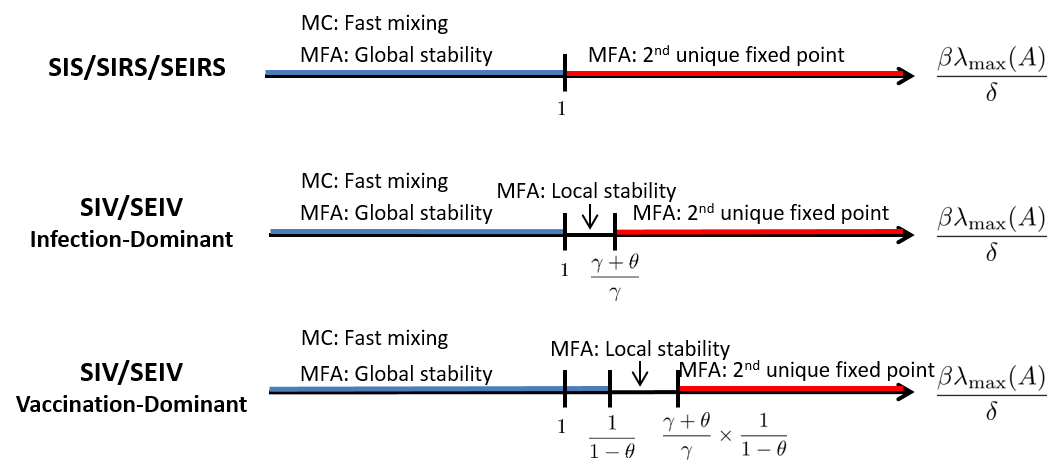# Navid Azizan## Analysis of Exact and Approximated Epidemic Models over Complex Networks

N. Azizan R., H. J. Ahn, and B. Hassibi

arXiv:1609.09565We consider the spread of discrete-time epidemics over arbitrary networks for well-known propagation models, namely SIS, SIRS, SEIRS, SIV, SEIV, and their variants. Such spreading processes can be normally described by Markov chains with an exponential number of states in the number of nodes. Since analyzing these Markov chain models is complicated, various linear and nonlinear lower-dimensional approximations of them have been proposed and studied in the literature. The most common of these is the nonlinear ‘‘mean-field’’ approximation and its linearization around the disease-free fixed point, whose number of states are linear in the number of nodes. We survey the prominent results on both the exact models and the approximated ones, with a focus on the connections between them. Since the linear model is the first-order approximation of the nonlinear mean-field one at the disease-free equilibrium, its stability determines the local stability of the nonlinear model. Furthermore, for most propagation models, the global dynamics of the nonlinear model also coincides with the stability of the linear model, and takes on one of two forms: either the epidemic dies out, or it converges to another unique fixed point (the so-called endemic state where a constant fraction of the nodes remain infected). These approximations are closely tied to the exact Markov chain by the fact that the linear model provides an upper-bound on the true marginal probabilities of infection, and that this is the tightest upper-bound that involves only marginals, in the ‘‘low-infection’’ regime. Furthermore, even though the nonlinear model is not an upper-bound on the true probabilities in general, it does provide an upper-bound on the probability of the chain not being in the all-healthy state. These bounds also imply the well-known result of sublinear mixing time of the Markov chain (epidemic extinction) when the disease-free fixed point is globally stable in the mean-field model. We compare these results for different propagation models in detail, and provide a concise summary of them.